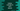# How can we print spaces in pythonPrinting spaces or blank spaces can be achieved in multiple ways in python. In this post, I will show you two different ways to print space in python with examples. Let’s have a look :

## Method 1: printing spaces in print() :

Simply, if you add any blank spaces in between a string, it adds that while printing :

``print("Hello World !!")``

It will print :

``Hello World !!``

Also, we can add spaces by using a empty print() statement :

``````print("Hello")
print()
print("World !!")``````

It will print :

``````Hello

World !!``````

Again, if we pass two params to print, it will add one blank space in between the words :

``````first_word = "Hello"
second_word = "World"

print(first_word,second_word)``````

It prints :

``Hello World``

## Method 2: Using a variable :

You can use one variable, if you want to have different amount of blank spaces in a text. For example :

``````space = ' '
first_word = "Hello"
second_word = "World"

print(first_word+space+second_word)
print(first_word+(space*2)+second_word)
print(first_word+(space*3)+second_word)
print(first_word+(space*4)+second_word)
print(first_word+(space*5)+second_word)``````

It will print :

``````Hello World
Hello  World
Hello   World
Hello    World
Hello     World``````

So, we can use one variable equal to a blank space and multiply it with any number to get a longer blank space.